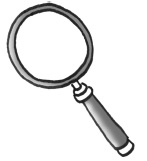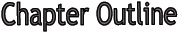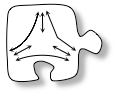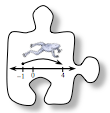# Chapter 3This chapter begins with a focus on multiple ways to represent portions, such as percents, decimals, and fractions.  You will use 100% blocks as a tool to explore the relationships between the equivalent representations of portions.  You will also connect portions to ratios.

In Section 3.2, you will describe how a point moves on a number line by adding integers.  You will also learn about absolute value and how to find the length of a line segment on a coordinate graph.

In this chapter, you will learn how to:

• Use a powerful new tool for finding equivalent fractions.
• Use percents, decimals, and fractions to describe a portion of a whole.
• Represent portions as percents, decimals, and fractions with pictures, symbols, and words.
• Find the decimal form of a number when it is given as a percent or fraction.
• Connect ratios to portions as ways to represent comparisons of parts
• Add positive and negative integers and rational numbers.
• Find the absolute value of a number.
• Find the length of horizontal and vertical line segments on a coordinate graph.

• ## Mathematical Vocabulary• The following is a list of vocabulary found in this chapter.  Some of the words have been seen in a previous chapter.  The italicized words are new to this chapter.  Make sure that you are familiar with the terms below and know what they mean.  Click on the word for a “pop-up” definition. For more information, refer to the glossary or index.  You might also add these words to your Toolkit so that you can reference them in the future.
•  absolute value coordinate graph decimal equivalent fractions equivalent ratios fraction greatest common factor integers least common multiple lowest terms multiplicative identity opposite ordered pair origin percent point portion prime factors quadrants ratio sample scale drawing simplify whole x-axis x-coordinate y-axis y-coordinateSection 3.1     In the first section, you will develop a useful tool for finding equivalent fractions and verifying that they are equivalent.  You will also represent portions of wholes as percents, decimals, and fractions.  Then you will work to find efficient ways to move between equivalent representations of the portions.Section 3.2     In this section you will describe motion on a number line using integers.  You will learn how addition can help you predict the starting or ending point of a series of moves.  Then you will relate this movement to finding distance.  Finally, you will connect your understanding of movement along a number line to distance on a coordinate graph.

Chapter 3 Focuses

Lessons:

Lesson 3.1.1

Lesson 3.1.2

Lesson 3.1.3

Lesson 3.1.4

Lesson 3.1.5

Lesson 3.1.6